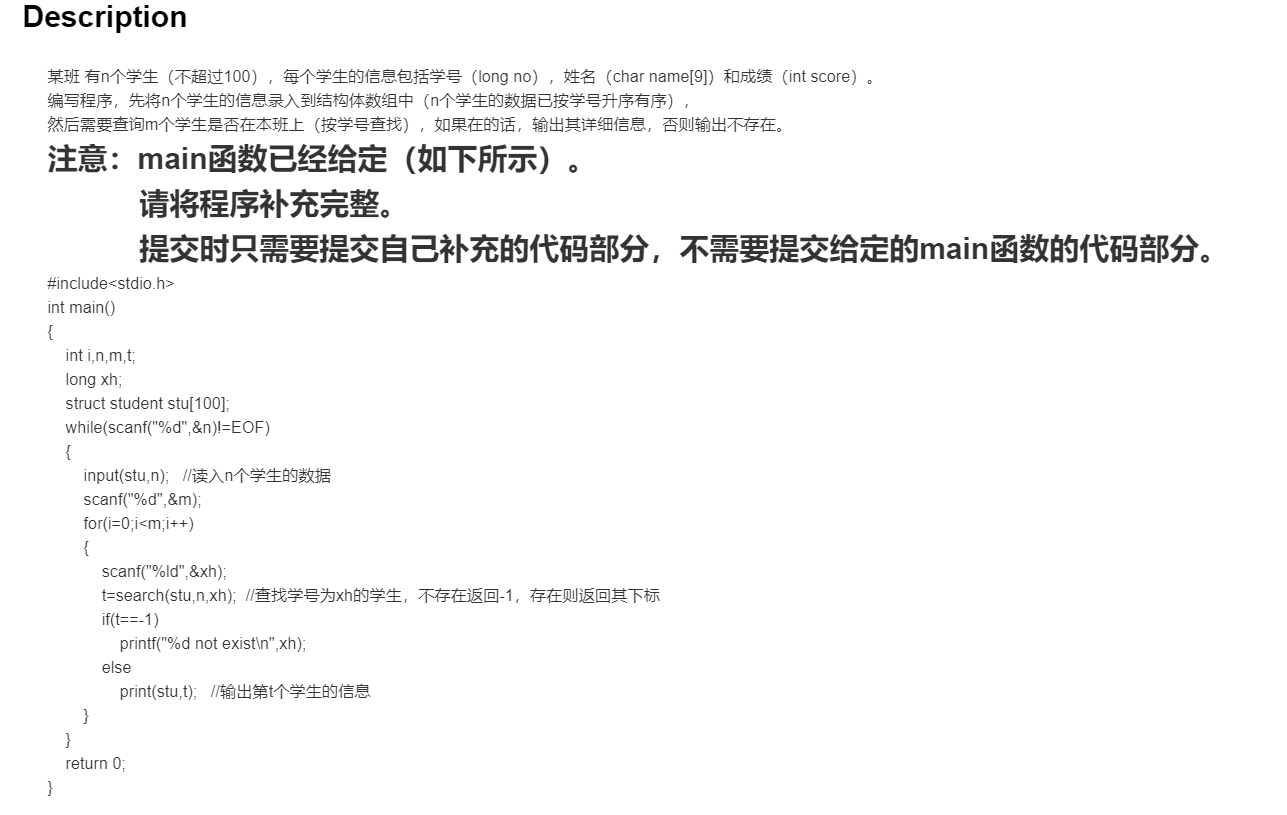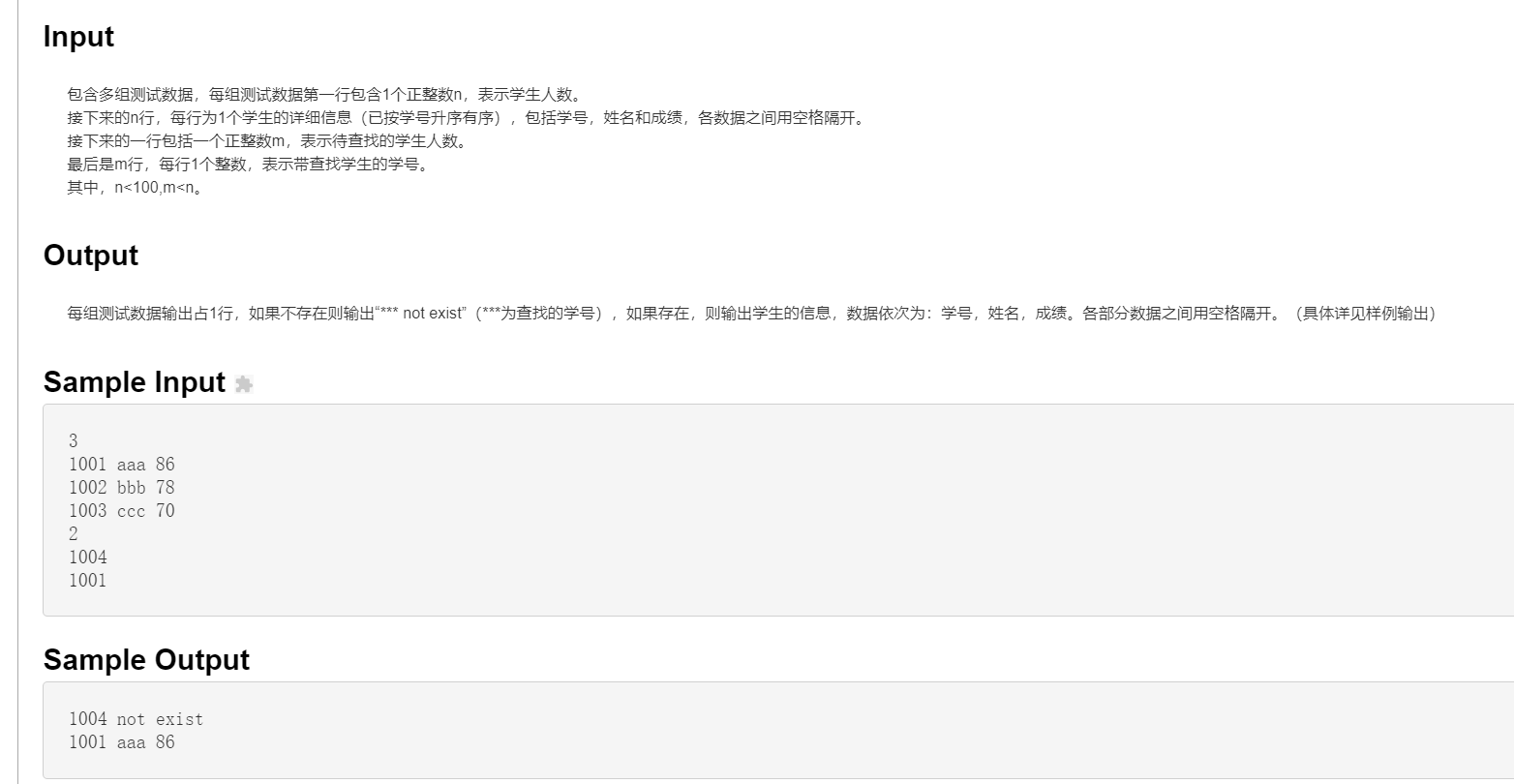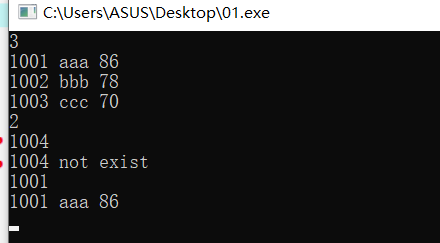2021-03-20 22:53

# 一道C++程序题，求大佬帮忙• 点赞
• 写回答
• 关注问题
• 收藏
• 复制链接分享
• 邀请回答

#### 4条回答

• ``````#include<stdio.h>

typedef struct student{
long no;
char name;
int score;
}Student;//为结构体类型struct student起别名Student

void input(Student stu[], int n){//读入n个学生的数据
for(int i = 0; i < n; i++){
scanf("%ld", &stu[i].no);
scanf("%s", stu[i].name);
scanf("%d", &stu[i].score);
}
}

//查找学号为xh的学生，不存在返回-1，存在则返回其下标
int search(Student stu[], int n, long no){
int index = -1;
for(int i = 0; i < n; i++){
if(no == stu[i].no){
index = i;
break;
}
}
return index;
}

void print(Student stu[], int t){//输出第t个学生的信息
printf("%ld %s %d\n", stu[t].no, stu[t].name, stu[t].score);
}

int main(){
int i, n, m ,t;
long xh;
struct student stu;
while(scanf("%d", &n) != EOF){
input(stu, n);//读入n个学生的数据
scanf("%d", &m);
for(i = 0; i < m; i++){
scanf("%ld", &xh);
t = search(stu, n, xh);//查找学号为xh的学生，不存在返回-1，存在则返回其下标
if(t == -1)
printf("%d not exist\n", xh);
else
print(stu, t);//输出第t个学生的信息
}
}
return 0;
}``````
点赞 评论 复制链接分享
• 题主多练习吧，没有尝试光问是不会熟记书写过程的。毫无经验可言

点赞 评论 复制链接分享
•点赞 评论 复制链接分享
• 自己作业自己做，手都没动，就想着白嫖！

点赞 评论 复制链接分享